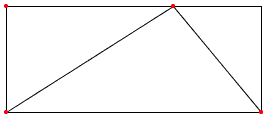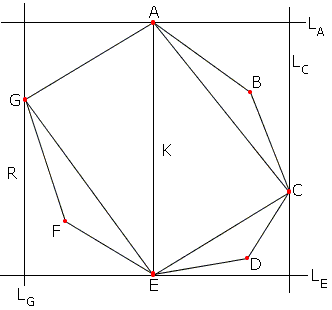# Polygon in a Rectangle

Every convex polygon of area 1 is contained in a rectangle of area 2.

ProofEvery convex polygon of area 1 is contained in a rectangle of area 2.

### Proof

The claim is certainly true for a triangle. Any triangle can be embedded in a rectangle of twice its area.Interestingly, the more general fact follows from this simple observation. Let K be a convex polygon of area 1. Pick two most distant vertices of the polygon.These are vertices A and E in the diagram. Through A and E draw lines LA and LE perpendicular to AE. Since the distance between A and E is the largest of all the distances between any two points of K, neither LA or LE meet K in points other than A and E. Draw a line perpendicular to LA and LE away from K on the right. Move towards K until it first touches K. The line will then share with K either a side of a vertex. In the diagram this is vertex C and the line is called LC. Similarly, find a line perpendicular to LA and LE to the left from K and move it towards K until the first contact. This is line LG in the diagram.

The four lines form a rectangle. Call it R. Line AE separates R into two smaller rectangles, say RG and RC.

Join A and E to C and G. The area of ΔAEG is half that of rectangle RG. The area of ΔAEC is half that of rectangle RC. The area of the quadrilateral ACEG is half that of R. Since K is convex, ACEG lies entirely within K and hence its area is at most 1. It follows that the area of R is at most 2. If need be, to answer the requirement it may be enlarged to enclose the area of 2.

### References

1. B. Bollobás, The Art of Mathematics: Coffee Time in Memphis, Cambridge University Press, 2006, p. 55.